NCERT Solutions for Class 10 Maths Chapter 12 Areas Related to Circles Ex 12.2 are part of NCERT Solutions for Class 10 Maths. Here we have given NCERT Solutions for Class 10 Maths Chapter 12 Areas Related to Circles Ex 12.2.

 Board CBSE Textbook NCERT Class Class 10 Subject Maths Chapter Chapter 12 Chapter Name Areas Related to Circles Exercise Ex 12.2 Number of Questions Solved 14 Category NCERT Solutions

## NCERT Solutions for Class 10 Maths Chapter 12 Areas Related to Circles Ex 12.2

Question 1.
Find the area of a sector of a circle with radius 6 cm if angle of the sector is 60°.
Solution:
Radius of the sector (r) = 6 cm
Central angle of the sector = 60°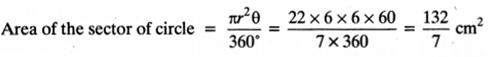Question 2.
Find the area of a quadrant of a circle whose circumference is 22 cm.
Solution:
Let radius of the circle = r
∴ Circumference of the circle = 2πr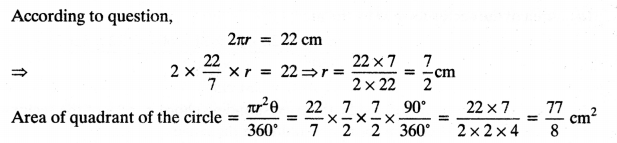Question 3.
The length of the minute hand of a clock is 14 cm. Find the area swept by the minute hand in 5 minutes.
Solution:
Length of minute hand of the clock = 14 cmQuestion 4.
A chord of a circle of radius 10 cm subtends a right angle at the centre. Find the area of the corresponding:
(i) minor segment
(ii) major segment (Use ? = 3.14)
Solution:
Given: radius of the circle = 10 cm
Angle subtended by chord at centre = 90°
(i) Area of the minor segment(ii) Area of the major segment = Area of the circle – Area of the minor segment
= πr2 – 28.5 = 3.14 x 10 x 10-28.5
= 314-28.5 = 285.5 cm2

Question 5.
In a circle of radius 21 cm, an arc subtends an angle of 60° at the centre. Find:
(i) length of the arc.
(ii) area of the sector formed by the arc.
(iii) area of the segment formed by the corresponding chord.
Solution:
Radius of the circle = 21 cm
Angle at the centre = 60°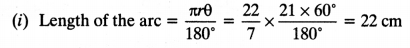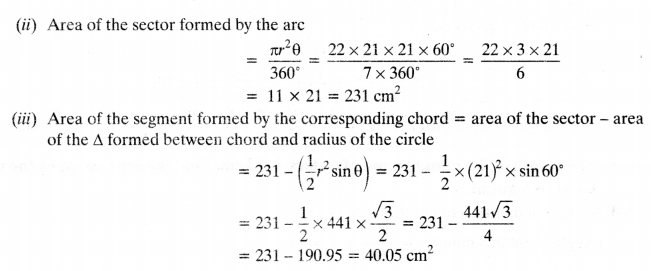Question 6.
A chord of a circle of radius 15 cm subtends an angle of 60° at the centre. Find the areas of the corresponding minor and major segments of the circle. (Use π = 3.14 and $$\sqrt{3}$$ = 1.73)
Solution:
Radius of the circle = 15 cm
Angle subtended by chord at centre = 60°
Area of the sector = $$\frac{\pi r^{2} \theta}{360^{\circ}}$$ = 3.14 x $$\frac{15 \times 15 \times 60^{\circ}}{360^{\circ}}$$ = 117.75 cm2
Area of the triangle formed by radii and chord = $$\frac { 1 }{ 2 }$$r2θ
= $$\frac { 1 }{ 2 }$$(15)2 sin 60° = $$\frac { 1 }{ 2 }$$ x 15 x 15 x $$\frac{\sqrt{3}}{2}$$ = 97.31 cm2
Area of the minor segment = Area of the sector – Area of the triangle formed by radii and chord
= 117.75 – 97.31 = 20.44 cm2
Area of the circle = πr2 = 3.14 x 15 x 15 = 706.5 cm2
Area of the circle – Area of the minor segment
= 706.5 – 20.44 = 686.06 cm2

Question 7.
A chord of a circle of the radius 12 cm subtends an angle of 120° at the centre. Find the area of the corresponding segment of the circle. (Use π = 3.14 and $$\sqrt{3}$$ = 1.73).
Solution:
Radius of the circle = 12 cm
Angle subtended by chord at centre = 120°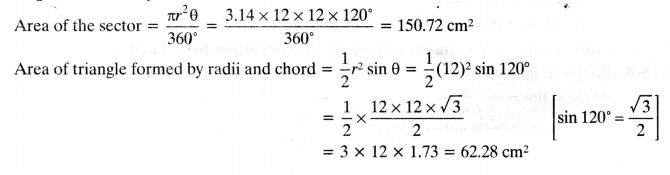Area of the corresponding segment = Area of the sector – Area of A formed by radii and chord
= 150.72 – 62.28 cm2 = 88.44 cm2

Question 8.
A horse is tied to a peg at one corner of a square shaped grass field of side 15 m by means of a 5 m long rope (see figure). Find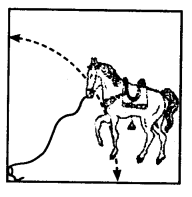(i) the area of that part of the field in which the horse can graze.
(ii) the increase in the grazing area if the rope were 10 m long instead of 5 m. (Use n = 3.14)
Solution:
(i) Length of the rope = Radius of the sector grazed by horse = 5 m
Here, angle of the sector = 90°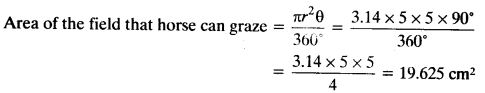Length of the rope is increased from 5 m to 10 m
New radius of sector grazed by horse = 10 m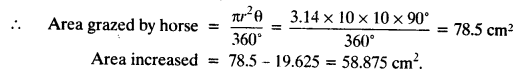Question 9.
A brooch is made with silver wire in the form of a circle with diameter 35 mm. The wire is also used in making 5 diameters which divide the circle into 10 equal sectors as shown in figure.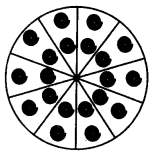Find:
(i) the total length of the silver wire required.
(ii) the area of each sector of the brooch.
Solution:
Length of one diameter = 35 mm
Total length of 5 diameters = 5 x 35 mm = 175 mm
Circumference of the circle = 2π = 2 x $$\frac { 22 }{ 7 }$$ x $$\frac { 35 }{ 2 }$$ = 110 mm
(i) Total length of the wire used = length of 5 diameters + circumference of brooch
= 175 + 110 = 285 mm

(ii) Total sectors are 10.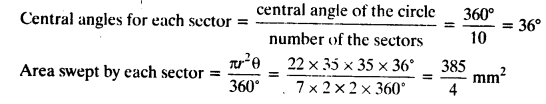Question 10.
An umbrella has 8 ribs which are equally spaced (see figure). Assuming umbrella to be a flat circle of radius 45 cm, find the area between the two consecutive ribs of the umbrella.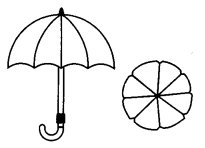Solution:
Radius of the circle = 45 cm
Number of ribs = 8Question 11.
A car has two wipers which do not overlap.
Each wiper has a blade of length 25 cm sweeping through an angle of 115°. Find the total area cleaned at each sweep of the blades.
Solution:
Area cleaned by each sweep of the blade = area of sector sweep by blade
Angle of the sector formed by blade of wiper =115°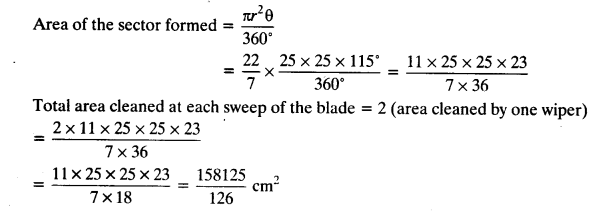Question 12.
To warn ships for underwater rocks, a lighthouse spreads a red coloured light over a sector of angle 80° to a distance of 16.5 km. Find the area of the sea over which the ships are warned. (Use π = 3.14)
Solution:
Angle of the sector = 80°
Distance covered = 16.5 km
Radius of the sector formed = 16.5 km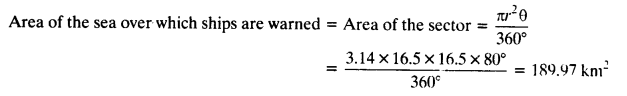Question 13.
A round table cover has six equal designs as shown in the figure. If the radius of the cover is 28 cm, find the cost of making the designs at the rate of ₹ 0.35 per cm2. (Use $$\sqrt{3}$$ = 1.7)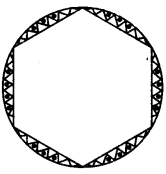Solution:
Radius of the cover = 28 cm
∵ There are six equal designs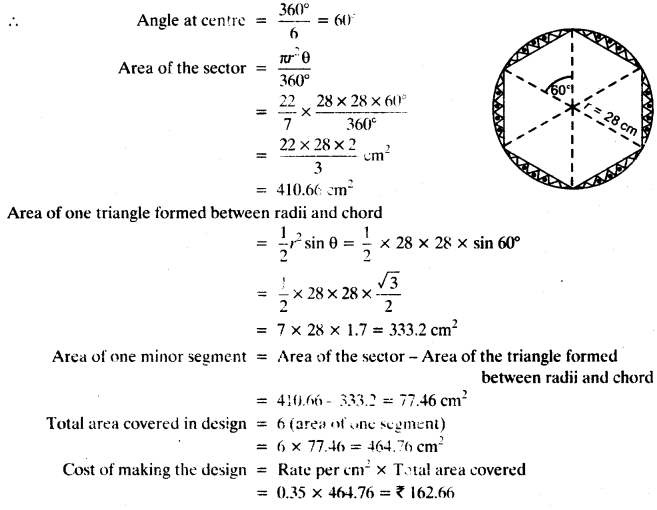Question 14.
Tick the correct answer in the following: Area of a sector of angle p (in degrees) of a circle with radius R is
(a) $$\frac{p}{180^{\circ}}$$ × 2πR
(b) $$\frac{p}{180^{\circ}}$$ × πR2
(c) $$\frac{p}{360^{\circ}}$$ × 2πR
(d) $$\frac{p}{720^{\circ}}$$ × 2πR2
Solution:
Sector angle isp in degrees
Radius of the circle = R
Area of the sector = $$\frac{\pi \mathrm{R}^{2} p}{361^{6}}$$ = $$\frac{\left(\pi R^{2} p\right) 2}{720^{\circ}}$$
= $$\frac{p}{720^{\circ}}$$ × 2πR2

We hope the NCERT Solutions for Class 10 Maths Chapter 12 Areas Related to Circles Ex 12.2, help you. If you have any query regarding NCERT Solutions for Class 10 Maths Chapter 12 Areas Related to Circles Ex 12.2, drop a comment below and we will get back to you at the earliest.